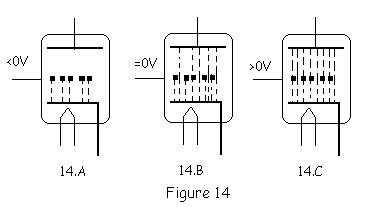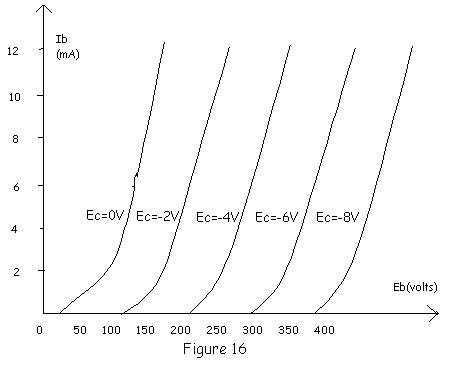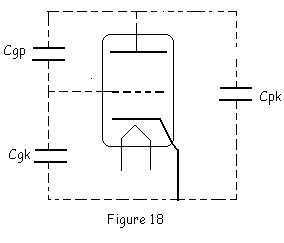www.SAP.or.id

3.Triode
In 1906 Dr Lee De Forest (1873-1961), an American scientist place the third electrode between cathode and plate of a vacuum diode. The resulting devices was called as triode.Simplified stucture and symbol of triode seen on Figure 13. As implies by its name triode have three electrodes, cathode, grid and plate.
Cathode stands on the centre surrounded by grid which is in the form of wire mesh. There is spaces between that wire mesh,  plate  cover both cathode and grid. Heated electron from cathode move to plate through the gap between those wires mesh.
In the real application of triode, grid controls the electron flow inside triode.
To get a clear idea about how control grid work let us see Figure 14When grid stands on negative voltage (Figure 14.A) or less than zero, the negative charge of grid will push back heated electron from cathode to emit and reach plate, so under this condition there is no electron emit from cathode and reach plate. Regarding to this situation there is a certain grid voltage that is to totally unabled heated electrons from cathode to emit and this grid voltage known as "grid cutt voltage"
When grid stands on zero volt (Figure 14.B) the heated electron from cathode start to emit and reach the plate. That's because zero voltage is still positive compare to negative charge of electron. Zero voltage is enough to attract heated electron to emit from cathode.
When grid stand on positive voltage (Figure 14.C) positive charge from grid will attract strongly heated electron from cathode to emit thorugh gap between wire mesh and reach plate. As grid positive voltage increase up, the amound of emitted electron will also increase up.

Note  : From now I will use Ec to represent grid voltage, Ib for plate current and EB for plate voltage.

*  Triode Characteristic
There are two important characteristic of triode, Plate Characteristic and Mutual Characteristic.
Test circuit to achieve those characteristic seen on figure 15 and resulting characterisctic seen on Figure 16 and Figure 17.On Figure 15 you'll find that there are two different variable voltage supply for grid and plate.
Grid voltage, Plate voltage and plate current measured by different meter. By this way both plate and mutual characteristic as seen on Figure 16 and 17 will achieve.
Plate Characteristic shows the coorelation bettwen plate voltage and the resulting plate current at the same grid voltage and Mutual Characteristic shows the coorelations between grid voltage and the resulting plate current at the same plate voltage. On the real application both Mutual and Plate characteristic are widely used to design tube electronic circuits.

*  vacuum Tube Constant
Tubes have there constant which are widely used in tube electronic design.
- Amplification factor (mu)
Mu indicates how sensitive grid voltage to control the changing of plate current.
Mu defined as the ratio of small changing in plate voltage to small changing in grid voltage at the same
plate current.

mu = d(Ep)/d(Ec)  ----> for d(Ib) = 0

- Transconductance  (gm)
Transkonductance indicates how sensitive grid voltage to influen plate current.
Transconductance defined as the ratio of small changing in plate current to small changing in plate
current.
gm = d(Ib)/d(Ec)  ----> for d(Eb)= 0

- Plate Resistance (rp)
Plate resistance is the ratio of small changing in plate voltage to small changing in plate current.
rp = d(Eb)/d(Ib)  ----> for d(Ec)= 0

-  Coorelation between mu gm and rp

mu =   d(Ep)/d(Ec) ...........................................................Eq 1

Multiply right side of the Eq 1 with 1 or d(Ib)/d(Ib) to make it become

mu = d(Ep)/d(Ec)  X  d(Ib)/d(Ib)................................Eq 2

Then modify Eq. 2  become

mu = d(Ib)/d(Ec)  X  d(Ep)/d(Ib).................................Eq 3

where d(Ib)/d(Ec) = gm     and     d(Ep)/d(Ib) = rp

Finally we can write Eq 3 as
mu = gm x rp ................................. Eq 4

* The Disadvantages of Triode
When the first time triode developed by Dr. Lee De Forest it gave many idea for further developement that never imagine before, but triode still have two disadvantages.
a) Amplification Factor (mu)
Triode's mu still  consider as not  high enough for many electronic applications
The highest mu I've ever found is 100, from 12AX7
b) Interelectrode capacitance between all the triode electrode make it become unsufficient for high
frequency application. Those interelectrode capacitance are Cgk ( between grid dan katoda) , Cgp
(between grid and plate), and Cpk (between  plate and cathode). Generally  value of the
interelectrode capacitance are in the range of 2 - 12 picofarad.
Especially for Cgp, on high frequency it will act as Miller Feedback capacitance that limit the high
frequency perfomance of the tube.Note About Triode Disadvantages
All the disadvantages I describe above relate to general application of triode, but in the case of Tube Audio Application, me and also many others tube lovers believe that triode is better compare to other tube. Triode perform  sweet sound compare to the others tube, to support my opinion about triode perfomance I  ask you  to see the trend of Tube Audio nowdays that many tube manufacture produce amplifier using triode, especially power amplifier using 300B direct heated triode.
You can forget about  Triode disadvantages for high frequency application because Audio amplifier only work up to maximum 20 kHz.